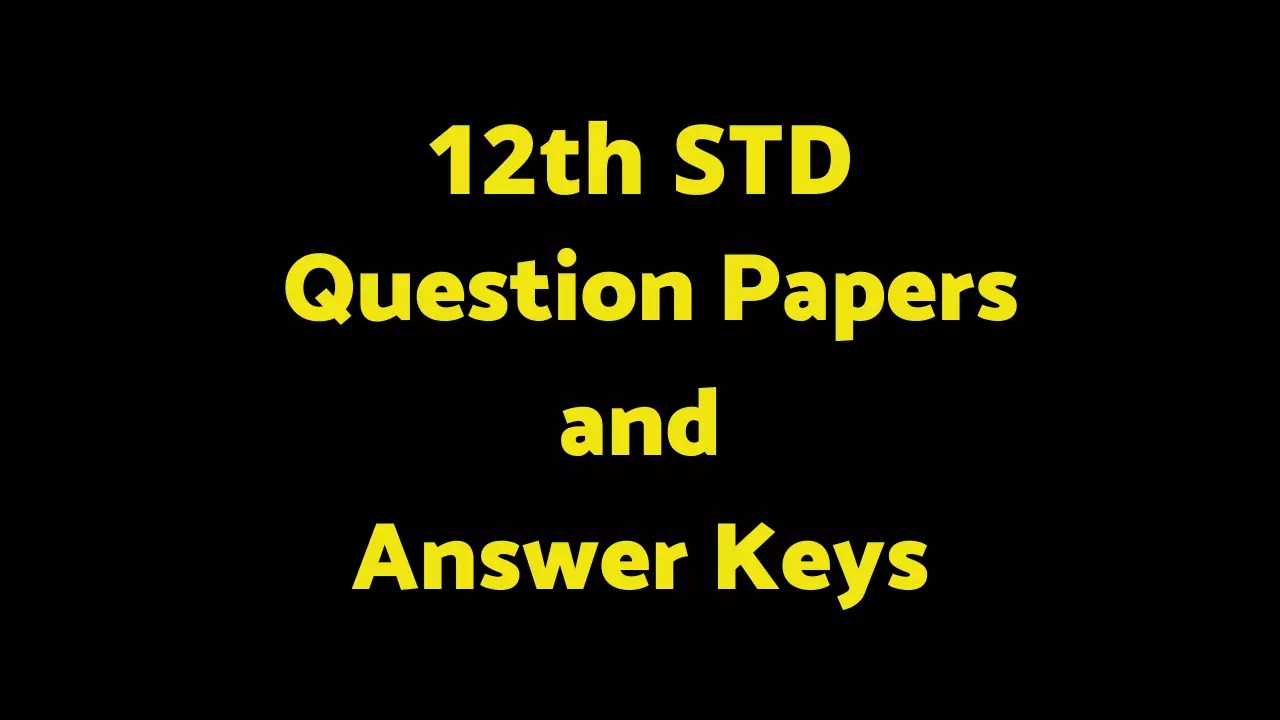# Model Question Paper 2021- 2022

## (November and December Month Syllabus)

12th COMPUTER SCIENCE Revision Test Model Question Paper 2021- 2022. TN 12th Standard Revision Test Model Question papers download PDF. 12th Samacheer kalvi Guide. 12th All Subject Unit Test All-District Question papers. 12th Revision Test Syllabus.• Time :03.00 hrs + 15 Minutes
• Maximum Marks:70

### Part – 1 (15 x 1= 15)

Note :

(ii) Choose the most suitable answer and write the code with the corresponding answer

1. The precondition of the function in algorithm is……………….
A) Requires
B) Returns
C) algorithm
D)subroutines

2. The small sections of code that are used to perform a particular task is called
A) Subroutines
B) Files
C) Pseudo code
D) Modules

3. Which of the following are mandatory to write the type annotations in the function definition?
A) Curly braces
B) Parentheses
C) S uare brackets
D) indentations

4. The process of providing only the essentials and hiding the details is known as
A) abstraction
B) Destruction
C) constructor
D) selectors

5. Which of the following functions that retrieve information from the data type?
A) Constructors
B) Selectors
C) recursive
D) Nested

6. The process of binding a variable ame with an object is called
A) Scope
B) Mapping
C) late binding
D) early binding

7. Which of the following is used • rogramming languages to map the variable and object?
A) ::
B) :=
C) =
D) ==

8. The way of defining an algorithm is called _
A) algorithmic strategy
B) algorithm
C) Feasibility
D) Input

9. …….also called sequential search is a sequential method for finding a particular value in a list.
A) Linear search
B) Binary search
C) Pseudo code
D) Bubble Search

10. From the following sorting algorithms which algorithm needs the minimum number of swaps?
A) Bubble sort
B) Quick sort
C) Merge sort
D) Selection sort

11. Two main measures for the efficiency of an algorithm are
A) Processor and memory
B) Complexity and capacity
C) Time and space
D) Data and space

12. The complexity of linear search algorithm is
A) O(n)
B) O(log n)
C) O(n2)
D) O(n log n)

A) www.python.org
B) www.tn.gov.in
D) www.sbionline.com

14. Who developed Python ?
A) Ritche
B) Guido Van Rossum
C) Bill Gates
D) Sunder Pitchai

15. Which operator is also called as Conditional operator?
A) Ternary
B) Relational
C) Logical
D) Assignment

## Part – 2 (6 x 2 = 12)

### Answer any six of the Following (Question No. 24 is compulsory)

16. What are the different operators that can be used in Python?
17. What are the different modes that can be used to test Python programs?
18. What is an Algorithm?
19. What is searching? Write its types.
20. What is Mapping?
21. Differentiate constructors and selectors.
22. What is a subroutine?
23. Differentiate interface and implementation.
24. Write the Output of the Below Program

## Part – 3 (6 x 3 = 18)

### Answer any six of the Following (Question No. 33 is compulsory)

25. Explain Ternary operator with examples.
26. Identify Which of the following are constructors and selectors?
(a) N l=number() (b) accetnum(nl) (c) displaynum(n l)
27. Define Global scope with an example.
28. Define Enclosed scope w”th an example.
29. List the characteristics of an algorithm.
30. Write short notes on Escape sequences with examples.
31. What are string lite als?- xplain.
32. Identify the scope of the variables in the following pseudo code and write its output
color:= Red
rnyco or():
b:=Blue
myfavcolor():
g:=Green
printcolor, b, g
myfavcolor()
printcolor, b
mycolor()
print color

33. Write the Output of the Below Program :-

## Part – 4 (5 x 5 = 25)

### Answer all the Questions : –

34. A) Explain the types of scopes for variable or LEGB rule with example.
[OR]
B) Discuss in detail about Tokens in Python.

35. A) Explain input() and print() functions with examples.
[OR]
B) Describe in detail the procedure Script mode programming.

36. A) Explain the characteristics of an algorithm.
[OR]
B) How will you facilitate data abstraction. Explain it with suitable e ample

37. A) Identify in the following program
let rec gcd a b :=
if b <> 0 then gcd b (a mod b) else return a
i) Name of the function
ii) Identify the statement which tells it is a recursive function
iii) Name of the argument variable
iv) Statement which fovoke the function recursively
v) Statement which te minates the recursion
[OR]
B) What are called Parameters and write a note on
(i) Parameter without Type (ii) Parameter with Type

38. A) Write your own python program using all Operators in Relational or Comparative operators
[OR]
B) Write a python program using any 5 of the Assignment operators in python

#### 12th COMPUTER SCIENCE Revision Test Model Question Paper 2021- 2022

 12th Revision Test Model Question Paper 2021-22

### This Post Has 5 Comments

1.Santhosh.S

Bro PDF file venum please send

2.muthu

ramnanathapuram districk computer science question

3.A. Karthikeyan

Sir pdf venum

4.A. Karthikeyan

Sir nenga super ra teach panringa enaku computer science and mathematics pannunga sir plz 🙏🙏

5.A. Karthikeyan

Computer science pdf send pannunga nalla teach pandringa tnx